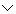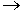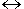# Challenge Exercises for Mathematical Logic

Directions: Read each question below. Create a truth table to help you answer each question. The answer choices provided correspond to the last column of the truth table for a given problem. Select your answer by clicking on its button. Feedback to your answer is provided in the RESULTS BOX. If you make a mistake, choose a different button.

 1. What are the truth values for this statement?   ~pq {T, F, F, F}{F, T, T, T}{F, F, T, F}None of the above. RESULTS BOX:
 2. What are the truth values for this statement?   p~q {T, T, F, T}{F, F, T, F}{F, T, F, F}None of the above. RESULTS BOX:
 3. What are the truth values for this statement?   ~ab {F, T, T, F}{T, T, T, F}{T, F, T, T}None of the above. RESULTS BOX:
 4. What are the truth values for this statement?   a~b {F, T, T, T}{T, F, T, F}{T, T, T, F}None of the above. RESULTS BOX:
 5. Choose the word or phrase that best completes this sentence:   The statements in problems 3 and 4 are ____________. Logically equivalentBiconditionalTautologiesNone of the above. RESULTS BOX:
 6. What are the truth values for this statement?   ~qp {F, T, T, T}{T, F, T, F}{T, T, T, F}None of the above. RESULTS BOX:
 7. Which statement below is logically equivalent to the statement in problem 6? pqpqpqNone of the above. RESULTS BOX:
 8. What are the truth values for this statement?   ~r~(rs) {T, T, T, T}{F, F, F, F}{T, T, T, F}None of the above. RESULTS BOX:
 9. What are the truth values for this statement?   (~qp)(pq) {F, T, T, T}{T, T, T, F}{T, T, T, T}None of the above. RESULTS BOX:
 10. Choose the word or phrase that best completes this sentence:   The statements given in problems 8 and 9 are _______. ConjunctionsTautologiesTriconditionalsNone of the above. RESULTS BOX:
 Lessons on Symbolic Logic Negation Conjunction Disjunction Conditional Compound Biconditional Tautologies Equivalence Practice Exercises Challenge Exercises Solutions